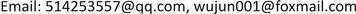1. 引言

2. 城镇化、产业结构与就业结构演变的描述性分析2.1. 山东省城镇化现状

Y = Y 1 Y 2 * 100 %  (1)

2.2. 产业结构与就业结构变化分析2.2.1. 三次产业GDP构成变化分析

2.2.2. 三次产业就业人数构成变化分析

2006~2016年山东省第一产业就业人员比重总体降低了10个百分点，2008年以来更是呈现出一种明显的持续下降趋势，从事第一产业的劳动人员有明显的减少，更多的劳动人员从事到第二、三产业的领域中。第二产业的就业人数呈现出曲折上升并且逐渐趋于较稳定的变化趋势，第二产业从业人员比重由2006年的31.4%增加到2016年的35.4%，总体的增加比重较小。第三产业从业人员的比重总体上升。

3. 城镇化水平对产业结构及就业结构影响的实证分析3.1. 相关性分析

3.1.1. 城镇化水平与三次产业产值构成相关关系

Correlation analysis of urbanization rate and output value of three industries in 2006 to 201

N11111111

N11111111

N11111111

N11111111
**在0.01水平(双侧)上显著相关

3.1.2. 城镇化水平与三次产业就业结构比重相关性分析

Correlation analysis of urbanization rate and employment proportion of three industries in 2006 to 201

N11111111

N9999

N9999

N9999
**在0.01水平(双侧)上显著相关

3.2. 回归模型分析

3.2.1. 城镇化水平与三次产业比重回归分析

G 1 = 19.140 − 0.198 * u , R 2 = 0.968 , F = 276.427 , t = − 16.626

G 2 = 105.081 − 1.011 * u , R 2 = 0.979 , F = 421.118 , t = − 20.521

G 3 = − 24.221 + 1.209 * u , R 2 = 0.979 , F = 413.968 , t = 20.346

3.2.2. 城镇化水平与三次产业就业结构回归分析

J 1 = 76.253 − 0.81 * u , R 2 = 0.981 , F = 468.482 , t = − 20.521

J 2 = 16.109 + 0.332 * u , R 2 = 0.917 , F = 99.366 , t = 9.968

J 3 = 7.639 + 0.477 * u , R 2 = 0.967 , F = 261.473 , t = 16.470

4. 结论与讨论